## 2010/03/31

### [C++] implicit constructor

 Ex1 class Base { public:     // 沒有定義constructor，則有一個implicitly constructor叫做: Base(){} }; class Derived: public Base { public: // Derived的constructor沒有呼叫其parent的constructor ，但compiler會implicitly的呼叫Base(){}。而由於Base(){} 也已經被compiler implicitly的定義了，所以沒問題。     Derived(int y)    {     } };

 Ex2 class Base { public:     Base(int x)    { // 已經有explicit constructor，則不會有implicit constructor     } }; class Derived: public Base { public: // 如果child class的constructor 沒有explicit 的呼叫parent的constructor，則compiler則會implicitly去呼叫其parent的implicit constructor，也就是沒有參數的constructor Base()。但是這個情況，Base()不會被宣告，所以會出現：「'Base' : 沒有適當的預設建構函式」的錯誤。     Derived(int y)    {      } };

 Ex3 class Base { public:     Base(int x)    { // 已經有explicit constructor，則不會有implicit constructor     } }; class Derived: public Base { public:     Derived(int y): Base(y)   {      } };

 Ex4 class Base { public:     Base(int x)    { // 已經有explicit constructor，則不會有implicit constructor     }     Base(){ }      // 因此我們主動宣告一個沒有參數的constructor }; class Derived: public Base { public:     Derived(int y):  {  // 這時候child 的constructor去implicitly的呼叫Base()就不會有錯誤了。     } };

## 2010/03/26

### [C++] 使用Pointer to pointer或Reference to pointer，改變pointer的address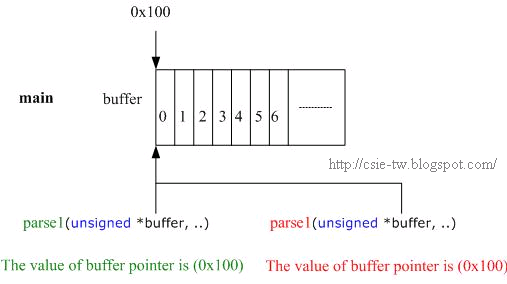一個錯誤的例子 #include using namespace std; #define BUFFER_SIZE 10 void readData(unsigned *buffer, int size) // Ex:從網路讀取資料寫道buffer {     for (int i = 0; i < size ; i++)    {         buffer[i] = i;     }    } void parse1(unsigned *buffer, int size) // 開始第一階段parse讀取到的資訊 {     for (int i = 0; i < size ; i++)    {         cout << "processing with parser1:" << *(buffer++) << endl; // 每讀取一個元素後，指標移動     }    } void parse2(unsigned *buffer, int size) // 希望從第一階段的parse後，繼續第二階段的parse {     for (int i = 0; i < size ; i++)    {         cout << "processing with parser2:" << *(buffer++) << endl;     }    } int main(void) {     unsigned buffer[BUFFER_SIZE];     readData(buffer, BUFFER_SIZE);     parse1(buffer,BUFFER_SIZE/2);     parse2(buffer,BUFFER_SIZE/2); // 此時buffer的pointer，並不會從一半開始，還是原本的頭開始     return 0;     /* output:     processing with parser1:0     processing with parser1:1     processing with parser1:2     processing with parser1:3     processing with parser1:4     processing with parser2:0     processing with parser2:1     processing with parser2:2     processing with parser2:3     processing with parser2:4     */   }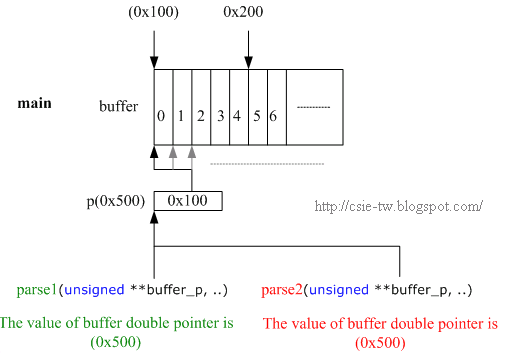一個正確的例子(pointer to pointer) #include using namespace std; #define BUFFER_SIZE 10 void readData(unsigned *buffer, int size) {     for (int i = 0; i < size ; i++)    {         buffer[i] = i;     }    } void parse1(unsigned **buffer_p, int size) {     for (int i = 0; i < size ; i++)    {         cout << "processing with parser1:" << *((*buffer_p)++) << endl;;     }    } void parse2(unsigned **buffer_p, int size) {     for (int i = 0; i < size ; i++)    {         cout << "processing with parser2:" << *((*buffer_p)++) << endl;;     }    } int main(void) {     unsigned buffer[BUFFER_SIZE];     unsigned *p = buffer;     readData(buffer, BUFFER_SIZE);     parse1(&p,BUFFER_SIZE/2);     parse2(&p,BUFFER_SIZE/2);     return 0;     /* output:      processing with parser1:0     processing with parser1:1     processing with parser1:2     processing with parser1:3     processing with parser1:4     processing with parser2:5     processing with parser2:6     processing with parser2:7     processing with parser2:8     processing with parser2:9     */ }

#### [C++] Call by value、Call by pointer、Call by reference

 一個正確的例子(reference to pointer) #include using namespace std; #define BUFFER_SIZE 10 void readData(unsigned *buffer, int size) {     for (int i = 0; i < size ; i++)    {         buffer[i] = i;     }    } void parse1(unsigned *&buffer, int size) {     for (int i = 0; i < size ; i++)    {         cout << "processing with parser1:" << *(buffer++) << endl;;     }    } void parse2(unsigned *&buffer, int size) {     for (int i = 0; i < size ; i++)    {         cout << "processing with parser2:" << *(buffer++) << endl;;     }    } int main(void) {     unsigned buffer[BUFFER_SIZE];     unsigned *p = buffer;     readData(buffer, BUFFER_SIZE);     parse1(p,BUFFER_SIZE/2);     parse2(p,BUFFER_SIZE/2);     return 0; /* output:     processing with parser1:0     processing with parser1:1     processing with parser1:2     processing with parser1:3     processing with parser1:4     processing with parser2:5     processing with parser2:6     processing with parser2:7     processing with parser2:8     processing with parser2:9     */ }

## 2010/03/22

### 敢找哈佛吵架的台大團隊

「Super Card」將所有代表的身分識別資訊、在台的食衣住行需求，整合在台北悠遊卡上，只要其中一項資訊更動，台大團隊建置的後勤支援資訊就會一併更新。

「就像是租借場地好了，我們真的很感謝台北市政府觀光傳播局，在這之前三個月我們至少碰了二十次釘子，完全沒有進展，還有的單位一下子說檔期可以，一下子說：『我們什麼時候答應你了？』」文化活動部門部長，台大中文系三年級的蘇煒翔說道。

「我們學會要找到關鍵人脈！」「還有動作要快，一旦關鍵人脈同意幫忙打電話，當下就牽上下一個人脈，把事情搞定。」侯宗成表示。

「就像是跨國企業母公司對子公司查核帳目一樣，」「對方直接拿出預算一條一條來談，哪些他們認為是有必要的，哪些沒有必要，要我們因應，而且口氣完全有話直說，沒有客氣寒暄，」財務部門部長、台大政治系三年級的虞雅惠感受到強烈的文化差異。

「我們要辦一場有史以來最棒的模聯活動！」這是台大團隊成員們最常提到的字眼。而過去這一年，為了完成這個夢想，這群年輕人在課業之外，每天投入平均五到六小時，籌辦會議，箇中的滋味，侯宗成最清楚。

「那時我們真的很怕接到他的電話，」呂艾珊描述，各部門被侯宗成追著寫企畫書；而且「他還會乾脆指示第一點、第二點怎麼做，很多人就覺得，啊，那你來就好啦！」宗成的戰友、台大國企系三年級的楊涵茜不客氣的吐槽。

「我的改變是被團隊逼出來的，很多時候，你要試著相信別人的善意，然後去試、去溝通，」他說，只有這樣最後才能協同合作。

### Convert.h

#ifndef CONVERT_H_
#define CONVERT_H_
#include <sstream>
#include <string>
using namespace std;
class Convert{
public:
static int str2int(const string &str);
static string int2str(const int n);
static double str2double(const string &str);
static string double2str(const double n);
};
#endif /*CONVERT_H_*/

### Convert.cpp

#include "Convert.h"
int Convert::str2int (const string &str) {
int n;
stringstream ss(str);
ss >> n;
return n;
};
string Convert::int2str (const int n) {
stringstream ss;
ss << n;
return ss.str();
}
double Convert::str2double(const string &str){
double n;
stringstream ss(str);
ss >> n;
return n;
}
string Convert::double2str(const double n){
stringstream ss;
ss << n;
return ss.str();
}

## 2010/03/21

### Dynamic Memory Allocation in C and C++ (malloc, new, and vector)

 1. C version - one dimension array #include // for malloc, realloc, free #include /* void * malloc ( size_t size ); - Allocates a block of size bytes of memory, returning a pointer to the beginning of the block. */ /* void free ( void * ptr ); - Deallocate space in memory previously allocated using a call to malloc, - calloc or realloc is deallocated, making it available again for further allocations. */ /* void * realloc ( void * ptr, size_t size ); - Reallocate memory block. - The content of the memory block is preserved up to the lesser of the new and old sizes. - If the new size is larger, the value of the newly allocated portion is indeterminate. */ int main() { int size = 10; // dynamically allocate a memory block with size x sizeof(int) int *arr = (int*)malloc(size * sizeof(int)); // the same as (int*) calloc (size, sizeof(int)); printf("address of arr is %X\n",arr); for (int i = 0; i < size ; i++){ arr[i] = 1; // Treat the memory block as a array } arr = (int*)realloc (arr ,2 * size * sizeof(int)); // Reallocate memory block printf("address of arr is %X\n",arr); for (int i = 0; i < 2*size ; i++){ printf("arr[%d]=%d\n",i,arr[i]); // arr[0~9] is 1. arr[10~19] is indeterminate. } free(arr); // Deallocate space return 0; }

 2. C version - two dimension array - pointer to pointer #include #include #include /* int **arr ----> |------|                       | int* | ---> | int int |                       | int* | ---> | int int |                       | int* | ---> | int int |                       |------|                                            ||                                            ||                                            ||                                           \/                                m x n 2-D array */ int main() { int m = 3 , n = 2; // m x n matrix int **arr; // pointer to pointer (double pointer) arr = (int**)malloc(m * sizeof(int*)); // sizeof(int*): the size of int pointer instead of int for (int i = 0; i < m ; i++) { arr[i] = (int*)malloc(n * sizeof(int)); // arr[i] is the same as *(arr+i). Ex: // *(arr+i) = (int*)malloc(n * sizeof(int)); } srand(time(NULL)); for (int i = 0; i < m ; i++) { for (int j = 0; j < n ; j++) { arr[i][j] = rand()%10; // Treat double pointer as 2-D array // *(*(arr+i)+j) = rand()%10; printf("%d\t",arr[i][j]); } printf("\n"); } // We should not forget to free the memory which we have allocated dynamically, // starting from the lowest level. i.e, in the reverse order of allocations. // If we free up the higher level pointers first the lower level pointers would be lost // and we would not be able to free up the memory allocated to those pointers. // This would lead to memory leaks. Given below is how we should free up the memory. for (int i = 0; i < m ; i++){ free(arr[i]); } free(arr); return 0; }

 3. C++ version - one dimension array #include #include #include using namespace std; // Type *array = new Type(size); // delete array; int main() { int size = 10; int *arr = new int[size]; // array with size = 10 int *p = new int(5); // a int with parameter 5. That is, *p is 5. for (int i = 0; i < size ; i++){ arr[i] = 1; // Treat the memory block as a array } /* Reallocate. - In contrast to C's realloc, it is not possible to directly reallocate memory allocated with new[]. - To extend or reduce the size of a block, one must allocate a new block of adequate size, - copy over the old memory, and delete the old block. - The C++ standard library provides a dynamic array that can be extended or reduced in its std::vector template. */ delete[] arr; // Arrays allocated with new[] must be deallocated with delete[]. delete p; return 0; }

 4. C++ version - two dimension array - pointer to pointer #include #include #include #include using namespace std; /* int **arr ----> |------|                       | int* | ---> | int int |                       | int* | ---> | int int |                       | int* | ---> | int int |                       |------|                                            ||                                            ||                                            ||                                           \/                                m x n 2-D array */ int main() { int m = 3 , n = 2; int **arr; arr = new int*[m]; for (int i = 0; i < m ; i++){ arr[i] = new int[n]; } srand(time(NULL)); for (int i = 0; i < m ; i++) { for (int j = 0; j < n ; j++){ arr[i][j] = rand()%10; cout << arr[i][j] << "\t"; } cout << endl; } for (int i = 0; i < m ; i++){ delete[] arr[i]; } delete[] arr; return 0; }

 5. C++ version - standard template library (STL) - vector - one and two dimension array #include #include #include using namespace std; int main() { /* Case #1 - 1D vector */ vector vector_1D; vector_1D.push_back(5); vector_1D.push_back(6); vector_1D.push_back(7); /* vector_1D has | int int int | */ for(int i=0;i<(int)vector_1D.size();i++) cout << vector_1D[i] << endl; vector_1D.clear(); // Actually, we don't need to delete allocated space. // Vector will delete it for us when vector_1D is out of the scope. /* Case #2 - 2D vector*/ int m=3, n=2; vector> vector_2D; vector_2D.resize(m); // there are m vector variables for (int i = 0; i < (int)vector_2D.size() ; i++){ vector_2D[i].resize(n); } /* vector_2D has | vector vector vector |                               ||                   ||                  ||                               ||                   ||                  ||                               \/                  \/                  \/                    has |int int|       has |int int|        has |int int| */ for (int i = 0; i < (int)vector_2D.size(); i++){ for (int j = 0; j <(int)vector_2D[i].size() ; j++){ vector_2D[i][j] = rand()%10; cout << vector_2D[i][j] << "\t"; } cout << endl; } return 0; }

### [C++] const的意義(const pointer, pointer to const, const pointer to a const, const function...)

#include<iostream>

#include<string>

using namespace std;

class A

{

private:

int y;

public:

A(){

y=0;

}

int f1(int x) const // 此const代表此function不可以修改Data Member

{

x++;

// y++; // Error! y為Data Member，因此不可以修改

return y;

}

const int f2(int x) // 回傳值為const

{

x++;

y++;

return y;

}

int f3(int const x) // 參數為const

{

// x++; // Error! x為const，不可以修改

y++;

return y;

}

void f4(int const *x) // x is variable pointer to a constant integer，也可以寫成f4(const int *x)

{

// (*x)++; // Error! pointer x所指向的實體為const，因此不可以修改其值

*x++; // 此行的意思是*(x++)，先修改pointer x的值，在指向實體

}

void f5(int const &x) // x is variable reference to a constant integer

{

// x++; // Error! x就是一個const

}

void f6(int *const x) // x constant pointer to a variable integer

{

(*x)++;

// *x++; // Error! Constant pointer x 不可以指向其他實體

}

const char * f7() // 回傳一個variable pointer to a constant char

{

return "ABC";

}

void f8(const int * const x) // x is constant pointer to a const integer

{

// (*x)++; // Error! x所指向的實體為const，因此不可以修改其值

// *x++; // Error! Constant pointer x 不可以指向其他實體

}

};

int main(){

A a;

int x=3;

a.f1(x);

a.f2(x);

a.f3(x); // int x 會自動轉成const int x傳入

a.f4(&x) ;

a.f5(x) ;

const char *s = a.f7(); // 必須使用一個const來接const

// s='0'; // Error! 無法對const 變數進行改變動作

return 0;

}

### [C++] Virtual Destructor

#include <iostream>

#include <string>

#include <vector>

using namespace std;

/*

1    *
Human -----> Tool
/\
|
|-----|
|      |
Pen   Pencil

*/

class Tool // the abstract class

{

public:

// no explicit constructor. there is a default one - Tool();

virtual void use() = 0;

virtual ~Tool(){

cout << "calling ~Tool()" << endl;

}

};

class Pen: public Tool

{

private:

int *x; // some private data;

public:

Pen(){

x = new int;

}

void use(){

cout << "using the Pen" << endl;

}

~Pen(){

cout << "calling ~Pen()" << endl;

delete [] x; // x is a pointer to dynamically allocated memory space in this object. Pencil is responsible to delete the space.

}

};

class Pencil: public Tool

{

private:

char *y; // some private data;

public:

Pencil(){

y = new char;

}

void use(){

cout << "using the Pencil" << endl;

}

~Pencil(){

cout << "calling ~Pencil()" << endl;

delete [] y; // y is a pointer to dynamically allocated memory space in this object. Pencil is responsible to delete the space.

}

};

class Human

{

private:

vector<Tool*> tools;

public:

void addTool(Tool* tool){ // keep the pointers to Tools only.

tools.push_back(tool);

}

void paint() // use each tool

{

for (int i = 0; i < (int)tools.size() ; i++){

tools[i]->use();

}

}

virtual ~Human()

{

cout << "Calling ~Human()" << endl;

for (int i = 0; i < (int)tools.size() ; i++){

delete tools[i]; // if the tools are not shared between other Human, this Human object should delete the tools.

}

}

};

int main()

{

Human *human = new Human();

human->paint();

delete human;

return 0;

}

/* output:

using the Pen

using the Pen

using the Pencil

Calling ~Human()

calling ~Pen()

calling ~Tool()

calling ~Pen()

calling ~Tool()

calling ~Pencil()

calling ~Tool()

*/

## 2010/03/20

### 台灣富人與外國窮人

【網路文章】有一回我到澳洲訪友，在居所附近的公園，遇上一個在河邊垂釣的中年男性。他很客氣主動與我招呼，我先是腆靦了一下，在台灣，陌生人的招呼是很不尋常的。他又主動問我從何處來，我說是台灣，他豎起大姆指說，那是一個富有的地方，他的熱情消除我的戒心，於是我們愉快地聊了起來。

「可是，沒錢花你快樂嗎？」我提出在我們國家失業的人必定的疑惑。「為什麼不快樂？我每天安排不同的娛樂，今天到河邊釣魚，明天到海邊抓蝦蟹，後天到山上採野果，這些都是免費的食物與享受，為什麼一定要花錢？難道你們國家不是這樣嗎？」

「不！在我們的國家沒有錢就沒有快樂，因為所有的快樂都是要花錢的，而且失業沒有救濟金，河裡釣不到魚，海裡抓不到蝦蟹，山上沒有野果，連公園裡的小水池都很髒，有錢人最大的快樂就是shopping，窮人就只能關在家裡看電視了。」我看著那隻生猛的魚沮喪說著。

「那麼你們賺的錢都花到那裡去？」他驚訝地問我。

## Call by value

int main() {
int x = 5;
foo(x);
}

void foo(int x) {
x++;
}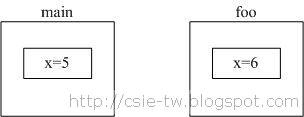## Call by pointer

int main() {
int x = 5;
foo(&x);
}

void foo(int *x) {
(*x)++; // 指向，並加1
}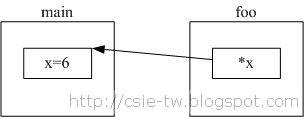## Call by reference

Call by reference的作用和目的和Call by pointer是一樣的，都是想要指回原本的變數並且可以修改。不過Call by reference寫起來更簡單。Ex:

int main() {
int x = 5;
foo(x); // 不用加&
}

void foo(int &x) {
x++; // 修改此x就是修改main的x
}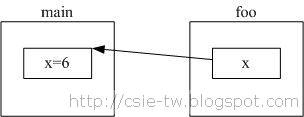int a = 10, b = 20;

int &x = a; // pointer x一開始就要固定指向實體，這時候是指向a，無法改變。

x++; // a變成11

int *y; // y是一個pointer，可以在一開始不指向任何實體。

y = &b; // y指向b

(*y)++; // b變成21

y = &a; // y改為指向a

(*y)++; // a變成12

## 2010/03/08

### Synergy：在多台電腦之間共享滑鼠鍵盤1. 下載並安裝synergy：http://sourceforge.net/projects/synergy2/files/

2. 右邊電腦設定如下

2.1 點選AutoStart，讓電腦可以自動開機執行此程式。

2.2 點選install即可開機執行。

2.3 接著點選「Configure」

2.4 新增2台電腦的Screen name：點選「+」

2.5 為2台電腦命名。比如「cs-right」代表左邊電腦，「cs-right」代表右邊電腦。

2.6 定義2台電腦之間的位子。在下方細紅框的地方選擇他們的關係，比如：

the cs-right of left goes to cs-left

the cs-left of right goes to cs-right

2.8 在screen name輸入：「cs-right」。

3. 左邊電腦設定如下

3.1 左邊電腦的角色為Client。在紅框1裡的Other computer's Host Name輸入：「cs-right」。

2.8 在screen name輸入：「cs-left」。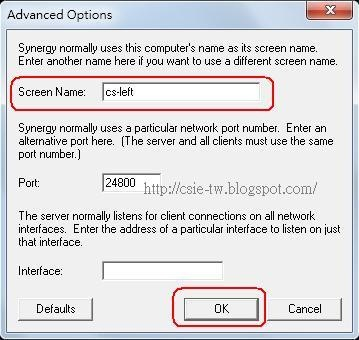2.9 最後按下「Start」即可。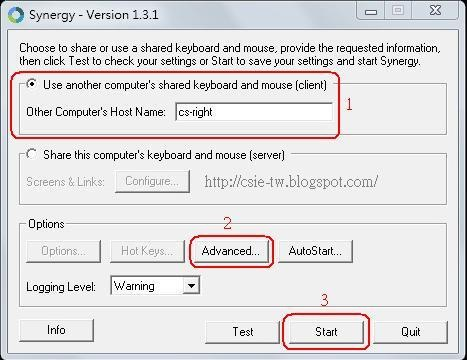### Hello Qt Creator(安裝與簡易視窗程式)

1. 安裝2. 建立新專案
2.1 開始Qt Creator2.2 File->New File or Project2.3 選擇Qt4 Gui Application2.4 輸入專案名稱和路徑。注意：路徑名稱只能為純英文。2.4 選擇會用到的模組2.5 設定主視窗名稱，使用預設即可。2.6 Finish2.7 點選Forms下的mainwindow.ui檔案，即可出現視窗介面。可以拖曳widget到window上。2.8 點選左下方的綠色箭頭按鈕，即可執行程式。## 2010/03/06

### 氣候紀錄片「正負2度C」：台灣恐成首批氣候難民

「目前全球平均溫度在十四．七度，已比一七五○年前高上○．八度。」汪中和指出，台灣早已成為氣候災民，莫拉克風災就是血淋淋的例子。如果全球溫度持續上升，海平面也會再升高，北冰洋一旦全融，會讓全球海洋升高六．六公尺，許多陸地將被淹沒。

±2°C」建議台灣參考荷蘭的因應措施。荷蘭新的國土規劃，預算規模超過國防預算，「還土於海」、「還土於河」，並致力研發水上房屋，準備好面對二○三五年海平面上升後的新地球生態。

±2°C」片中呈現中央氣象局資料，把台灣從一九四七到一九九六年間的每一條襲台颱風路徑畫成一條直線，台灣像是一塊被竹編包得密不透風的土地，雨量最密集地區就落在花蓮與阿里山以下的中南部地區。去年莫拉克襲台兩個月後，台灣最北端的翡翠灣，居然佈滿來自中部山區及阿里山區的樹木，山崩樹倒、山林遷徙，顯示「氣候的力量，超越戰爭」。

±2°C」不選擇於全台戲院放映，主要是考慮民眾到戲院觀看會帶來更多碳排放足跡，台灣的多家電視台，包括緯來、Discovery Channel、慈濟大愛、人間衛視及中華電信MOD等頻道，都已加入無償播放紀錄片行列。該片也能在官方網站（http://正負2度c.tw ）讓民眾免費下載。

### 台灣好行：後慈湖、大溪老街半日遊